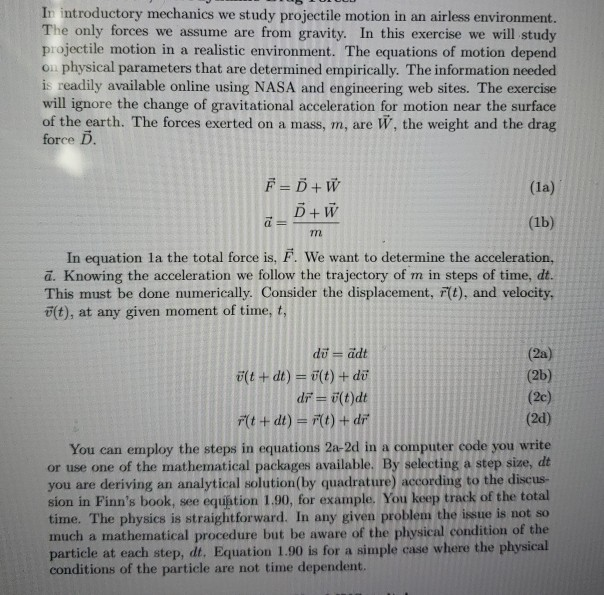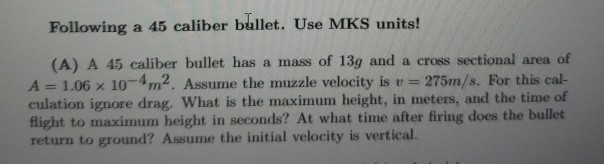# (Solved) : Read Following Information Create Python Code Following Situation Muzzle Velocity Initial Q42746274 . . .To create a python code for the followingsituationWhere the muzzle velocity is initial velocity

In introductory mechanics we study projectile motion in an airless environment. The only forces we assume are from gravity. In this exercise we will study projectile motion in a realistic environment. The equations of motion depend on physical parameters that are determined empirically. The information needed is readily available online using NASA and engineering web sites. The exercise will ignore the change of gravitational acceleration for motion near the surface of the earth. The forces exerted on a mass, m, are W, the weight and the drag force D. (la) F=Õ + W B + W (1b) In equation la the total force is, F. We want to determine the acceleration, ā. Knowing the acceleration we follow the trajectory of m in steps of time, dt. This must be done numerically. Consider the displacement, F(t), and velocity, öſt), at any given moment of time, t, dü = ädt ült + dt) = u(t) + dü di = 8(t)dt Ft + dt) = F(t) + dr (20) You can employ the steps in equations 2a-2d in a computer code you write or use one of the mathematical packages available. By selecting a step size, dt you are deriving an analytical solution(by quadrature) according to the discus- sion in Finn’s book, see equation 1.90, for example. You keep track of the total time. The physics is straightforward. In any given problem the issue is not so much a mathematical procedure but be aware of the physical condition of the particle at each step, dt. Equation 1.90 is for a simple case where the physical conditions of the particle are not time dependent. Following a 45 caliber bullet. Use MKS units! (A) A 45 caliber bullet has a mass of 13g and a cross sectional area of A = 1.06 x 10-4m2. Assume the muzzle velocity is v = 275m/s. For this cal- culation ignore drag. What is the maximum height, in meters, and the time of flight to maximum height in seconds? At what time after firing does the bullet return to ground? Assume the initial velocity is vertical. Show transcribed image text In introductory mechanics we study projectile motion in an airless environment. The only forces we assume are from gravity. In this exercise we will study projectile motion in a realistic environment. The equations of motion depend on physical parameters that are determined empirically. The information needed is readily available online using NASA and engineering web sites. The exercise will ignore the change of gravitational acceleration for motion near the surface of the earth. The forces exerted on a mass, m, are W, the weight and the drag force D. (la) F=Õ + W B + W (1b) In equation la the total force is, F. We want to determine the acceleration, ā. Knowing the acceleration we follow the trajectory of m in steps of time, dt. This must be done numerically. Consider the displacement, F(t), and velocity, öſt), at any given moment of time, t, dü = ädt ült + dt) = u(t) + dü di = 8(t)dt Ft + dt) = F(t) + dr (20) You can employ the steps in equations 2a-2d in a computer code you write or use one of the mathematical packages available. By selecting a step size, dt you are deriving an analytical solution(by quadrature) according to the discus- sion in Finn’s book, see equation 1.90, for example. You keep track of the total time. The physics is straightforward. In any given problem the issue is not so much a mathematical procedure but be aware of the physical condition of the particle at each step, dt. Equation 1.90 is for a simple case where the physical conditions of the particle are not time dependent.
Following a 45 caliber bullet. Use MKS units! (A) A 45 caliber bullet has a mass of 13g and a cross sectional area of A = 1.06 x 10-4m2. Assume the muzzle velocity is v = 275m/s. For this cal- culation ignore drag. What is the maximum height, in meters, and the time of flight to maximum height in seconds? At what time after firing does the bullet return to ground? Assume the initial velocity is vertical.

Answer to Read the following information To create a python code for the following situation Where the muzzle velocity is initial …

We are the best freelance writing portal. Looking for online writing, editing or proofreading jobs? We have plenty of writing assignments to handle.